Next: Spin Precession Up: Spin Angular Momentum Previous: Rotation Operators in Spin

# Magnetic Moments

Consider a particle of electric charge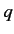and speed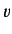performing a circular orbit of radiusin the-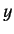plane. The charge is equivalent to a current loop of radiusin the-plane carrying current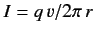. The magnetic moment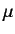of the loop is of magnitude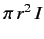and is directed along the-axis. Thus, we can write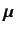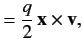(458)

where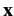and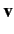are the vector position and velocity of the particle, respectively. However, we know that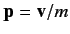, where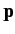is the vector momentum of the particle, and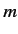is its mass. We also know that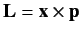, where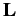is the orbital angular momentum. It follows that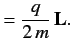(459)

Using the usual analogy between classical and quantum mechanics, we expect the above relation to also hold between the quantum mechanical operators,and, which represent magnetic moment and orbital angular momentum, respectively. This is indeed found to the the case.

Spin angular momentum also gives rise to a contribution to the magnetic moment of a charged particle. In fact, relativistic quantum mechanics predicts that a charged particle possessing spin must also possess a corresponding magnetic moment (this was first demonstrated by Dirac--see Chapter 11). We can write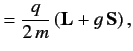(460)

where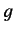is called the gyromagnetic ratio. For an electron this ratio is found to be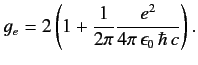(461)

The factor 2 is correctly predicted by Dirac's relativistic theory of the electron (see Chapter 11). The small correction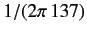, derived originally by Schwinger, is due to quantum field effects. We shall ignore this correction in the following, so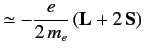(462)

for an electron (here,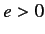).Next: Spin Precession Up: Spin Angular Momentum Previous: Rotation Operators in Spin
Richard Fitzpatrick 2013-04-08